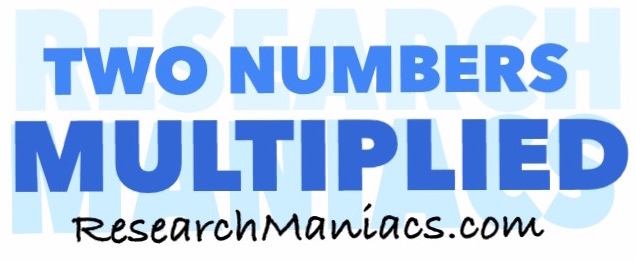What two numbers multiply to 8?What 2 numbers do you multiply to get 8? In other words, what number can you multiply with another number to get 8?

The formula to solve this would be:

X x Y = 8

There are at least two combinations of two numbers that you can multiply together to get 8.

For your convenience, we have made a list of all the combinations of two numbers multiplied by each other that will make 8:

1 x 8 = 8
2 x 4 = 8
4 x 2 = 8
8 x 1 = 8

Two Numbers Multiplied
Enter another number below to see which combinations of two numbers multiplied will equal that number.

What two numbers multiply to 9
Go here for the next number on our list.